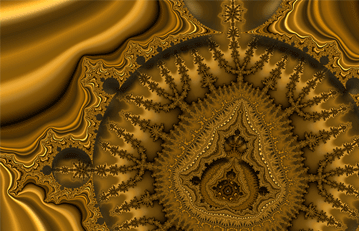2.3 - Mathematical FractalsHandsOn Activities:

8. Creating Your Own Mathematical Fractal

We learned previously that there are two types of fractals: natural and mathematical. Unlike a natural fractal, a mathematical fractal is constructed according to a set of fixed rules which do not involve any random processes. Given the fixed rules, the resulting structures are always identical to one another.

Now we create a coastline that is a mathematical fractal, using a different set of rules-rules that have no randomness. You won't be needing any dice to throw or coins to flip. Since the rules have no randomness, this time you and your classmates should get the identical result.

Next: HandsOn 8- Creating Your Own Mathematical Fractal